# Previous Year Questions: Magnetic Effects of Current Notes | Study Science Class 10 - Class 10

## Class 10: Previous Year Questions: Magnetic Effects of Current Notes | Study Science Class 10 - Class 10

The document Previous Year Questions: Magnetic Effects of Current Notes | Study Science Class 10 - Class 10 is a part of the Class 10 Course Science Class 10.
All you need of Class 10 at this link: Class 10

Q.1. Two circular coils P and Q are kept close to each other, of which coil P carries a current. What will you observe in the galvanometer connected across the coil Q,
(a) if current in the coil P is changed?
(b) if both the coils are moved in the same direction with the same speed?

Give reason to justify your answer in each case.     [CBSE 2011, 2019] [2 marks]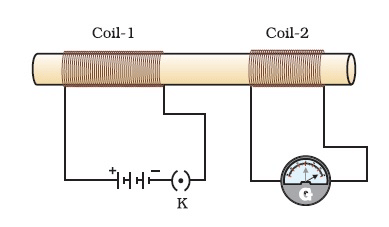Ans. (a) If current in the coil P [Fig. 13.24] is increased, we get momentary deflection in galvanometer connected across the coil Q in one direction. However, if current in the coil P is decreased, the deflection in galvanometer is in opposite direction. Due to change of current in coil P, the magnetic field of coil Q also changes and so as induced current is set up in coil Q and the galvanometer shows a deflection.
(b) If both the coils P and Q are moved in the same direction with the same speed, the magnetic field of both the coils remain unchanged. Hence no induced current is set up in coil Q and there is no deflection in the galvanometer.

Q.2. What is the function of a galvanometer in a circuit?    [CBSE 2019] [1 mark]
Ans. A galvanometer is used to detect the flow of current, if any, in a circuit.

Q.3. One of the major causes of fire in office buildings is short-circuiting. List three reasons which may lead to short-circuiting. How can it be prevented?    [CBSE2016] [3 marks]
Ans. Three possible reasons of short-circuiting of an electrical circuit are as follows:

• The insulation of electrical wirings is damaged.
• The electrical appliance used in the circuit is defective.
• An appliance of higher power rating is being run on an electrical line of lower power rating.

Short-circuiting can be prevented by the use of electrical fuse of appropriate capacity.

Q.4. What is an electric fuse ? Briefly describe its function.    [CBSE 2010,2011,2012,2013]
Or

Explain the use of electrical fuse. What type of fuse material is used for fuse wire and why?    [CBSE 2013,2014,2016]  [2 marks]
Ans. An electric fuse is a device that is used ahead of and in series of an electric circuit as a safety device to prevent the damage caused by short-circuiting or overloading of the circuit.
It is a small, thin wire of a material whose melting point is low. Generally, wire of tin or tin-lead alloy or tin-copper alloy is used as a fuse wire. If due to some fault electric circuit gets short-circuited, then a strong current begins to flow. Due to such a strong flow of current, the fuse wire is heated up and gets melted. As a result, the electric circuit is broken and current flow stops. Thus, possible damage to the circuit and appliances is avoided.

Q.5. (a) List four factors on which the magnitude of magnetic force acting on a moving charge in a magnetic field depends.
(b) How will a fine beam of electrons streaming in west to east direction be affected by a magnetic field directed vertically upwards? Explain with the help of a diagram mentioning the rule applied.     [CBSE 2014, 2016]  [3 marks]
Ans. (a) The magnitude of the magnetic force acting on a moving charge in a magnetic field depends on:

• the magnitude of charge
• speed of moving charge
• strength of magnetic field
• the angle between the direction of motion of charge and the direction of magnetic field.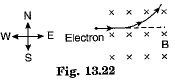(b) The electron streaming from west to east is equivalent to a current from east to west. The magnetic field B is vertically upwards and shown by cross marks (x). Hence, in accordance with Fleming’s left-hand rule, the electron will experience a force in north direction and deflected in that direction.

Q.6. What is a solenoid? Draw magnetic field lines due to a current-carrying solenoid. Write three important features of the magnetic field obtained.     [CBSE 2010, 2011, 2012, 2014, 2015, 2016] [3 marks]
Ans. A solenoid is a coil of large number of circular turns of wire wrapped in the shape of a cylinder. On passing electric current, a magnetic field is developed. Magnetic field lines are drawn below. The field is along the axis of solenoid such that one end of solenoid behaves as north pole and the other south pole. Thus, field of a solenoid is similar to that of a bar magnet.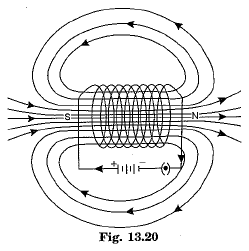Important features of magnetic field due to a current-carrying solenoid are:

• Magnetic field lines inside the solenoid are nearly straight and parallel to its axis. It shows that magnetic field inside a solenoid is uniform.
• Magnetic field of solenoid is identical to that due to a bar magnet with one end of solenoid behaving as a north pole and other end as a south pole.
• A current-carrying solenoid exhibits the directive and attractive properties of a bar magnet.

Q.7. Describe an activity with a neat diagram to demonstrate the presence of magnetic field around a current-carrying straight conductor.    [CBSE 2010, 2012, 2014, 2016] [3 marks]
Ans.

• Take a long straight thick copper wire and insert it through the centre of a plain cardboard. Cardboard is fixed so that it is perfectly horizontal and the thick wire is in vertical direction.
• Prepare an electric circuit consisting of a battery, a variable resistance, an ammeter and a plug key.
• Connect the copper wire in the circuit between the points X and Y. Sprinkle some iron filings uniformly on the cardboard. Put the plug in key K so that a current flows in the circuit.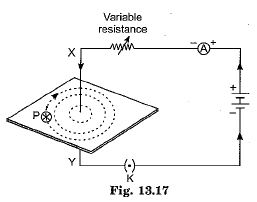• Gently tap the cardboard using a finger. We observe that the iron filings align themselves forming a pattern of concentric circles around the copper wire.
• These concentric circles represent the magnetic field lines. Direction of field lines is determined by the use of a compass needle. If current is flowing vertically downward then the magnetic field lines are along clockwise direction. If current is flowing vertically upward then the field lines are along anticlockwise direction.

Q.8. (a) Describe an activity to draw a magnetic field line outside a bar magnet from one pole to another pole.    [CBSE 2012, 2014, 2016]
(b) What does the degree of closeness of field lines represent? [3 marks]
Ans. (a) Take a bar magnet and place it on a sheet of white paper fixed on a drawing sheet. Mark the boundary of magnet. Take a small compass and place it near the north pole of the magnet. Mark the position of two ends of compass needle. Move the needle to a new position so that its south pole occupies the position previously occupied by its north pole. In this way proceed step by step till we reach the south pole of magnet. Join the points marked on the paper by a smooth curve. This curve represents a magnetic field line as shown in Fig. 13.15.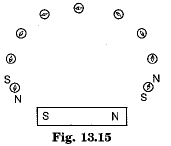(b) The degree of closeness of magnetic field lines signifies the strength of magnetic field.

Q.9. What type of current is given by a cell?    [CBSE 2011, 2012, 2014, 2016]  [1 mark]
Ans. Direct current (D.C.).

Q.10. Write any one method to induce current in a coil.    [CBSE 2014, 2016]  [1 mark]
Ans. Whenever magnetic field around a coil is changed, a current is induced in the coil.

Q.11. Under what condition is the force by a current-carrying conductor placed in a magnetic field maximum?    [CBSE 2009, 2012, 2016] [1 mark]
Ans. The force acting on a current-carrying conductor placed in a magnetic field is maximum when the direction of current is at right angles to the direction of the magnetic field.

Q.12. Define magnetic field of a bar magnet.     [CBSE 2006,2010,2014,2015,2016]  [1 mark]
Ans. The magnetic field of a bar magnet is the region around the magnet in which force due to magnet can be felt.

Q.13. What is the shape of magnetic field lines due to a straight current-carrying conductor?    [CBSE 2010,2013,2014,2015]  [1 mark]
Ans. Magnetic field lines form concentric circles around the conductor with conductor at the centre.

Q.14. What are magnetic field lines?    [CBSE 2010,2011,2012,2015]  [1 mark]
Ans. A magnetic field line around a magnet is the path along which north pole of a magnetic compass needle points. A magnetic field line gives the direction of magnetic field at a point.

Q.15. (a) Describe an activity to show that an electric current-carrying wire behaves like a magnet.
(b) Write the rule which determines the direction of magnetic held developed around a current-carrying straight conductor.    [CBSE 2010, 2011, 2012, 2014, 2015] [3 marks]

Ans. (a) Take a straight, thick copper wire X Y and connect it to an electric circuit consisting of a battery, key and resistor. Place a small compass near the copper wire. Now put the plugin key K so that a current begins to flow. The compass needle is deflected.
It shows that the current-carrying copper wire is behaving like a magnet.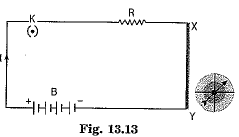(b) The direction of the magnetic field around a straight current-carrying conductor is given by the “right-hand thumb rule”. According to the rule, imagine holding the current-carrying straight conductor in your right hand such that the thumb points towards the direction of the current. Then the fingers of the right hand wrap around the conductor in the direction of the field lines of the magnetic field.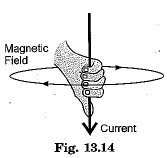Q.16. Out of the three wires live, neutral or earth, which one goes through ON/ OFF switch?    [CBSE 2010, 2012, 2014, 2015]  [1 mark]
Ans. The live wire.

Q.17. Why does a current-carrying conductor experience a force when it is placed in a magnetic field?    [CBSE 2011, 2015] [1 mark]
Ans. A current-carrying conductor produces a magnetic field around it. This magnetic field interacts with the externally applied magnetic field and as a result the conductor experiences a force.

Q.18. What is the frequency of A.C. being supplied in our houses?    [CBSE 2010, 2013, 2015]

[1 mark]
Ans. 50 Hz.

Q.19. Distinguish between a direct current and an alternating current.    [CBSE 2012, 2013, 2014, 2015] [3 marks]
Ans.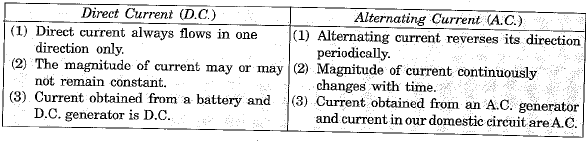Q.20. Describe an activity to explain how a moving magnet can be used to generate electric current in a coil.    [CBSE 2012,2014,2015]
Or
A coil made of insulated copper wire is connected to a galvanometer. What will happen to the deflection of the galvanometer if a bar magnet is pushed into the coil and then pulled out of it? Give reason for your answer and name the phenomenon involved.    [CBSE 2010,2011,2012] [3 marks]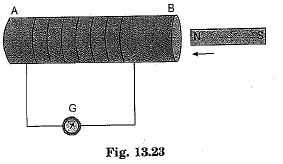Ans. Take a coil AB of insulated copper wire having a number of turns. Connect the ends of coil to a sensitive galvanometer G. Now take a bar magnet NS and rapidly bring the magnet towards the end B of coil as shown in Fig. 13.23.

The galvanometer gives momentary deflection in one direction. Now take the magnet away from the coil, the galvanometer again gives momentary deflection but in the opposite direction. It clearly shows that motion of magnet induces, a current in the coil.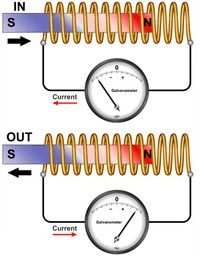Again keep the magnet fixed and gently move the coil AB either towards the magnet or away from the magnet. We get deflection in the galvanometer even now. Thus, an induced current is produced in a coil whenever there is relative motion between the coil and the magnet. This phenomenon is known as electromagnetic induction.

Q.21. A metallic conductor is suspended perpendicular to the magnetic field of a horse-shoe magnet. The conductor gets displaced towards left when a current is passed through it. What will happen to the displacement of the conductor if the :
(i) current through it is increased?
(ii). horse-shoe magnet is replaced by another stronger horse-shoe magnet?
(iii) direction of current through it is reversed ?     [CBSE 2011, 2012, 2015] [3 marks]
Ans. (i) On increasing the current flowing through metallic conductor, the force experienced by it is proportionately increased because F ∝ I.
(ii) On using a stronger horse-shoe magnet the magnetic force increases because F
(iii) On reversing the direction of current the direction of force is reversed and conductor is displaced towards right instead of left direction.

Q.22. For the current carrying solenoid as shown below, draw magnetic field lines and giving reason explain that out of the three points A, B and C at which point the field strength is maximum and at which point it is minimum.    [CBSE  2015] [2 marks]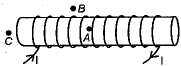Ans. Outside the solenoid magnetic field is minimum. At the ends of solenoid, magnetic field strength is half that of inside it. So Minimum - at point B; Maximum - at point A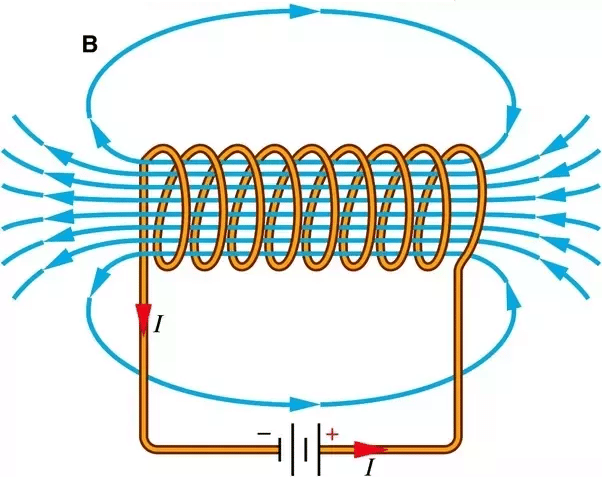Q.23. What is meant by solenoid? How does a current carrying solenoid behave? Give its main use.    [CBSE 2015] [3 marks]
Ans. Solenoid: A coil of many circular turns of insulated copper wire wound on a cylindrical insulating body (i.e. cardboard etc.) such that its length is greater than its diameter is called solenoid.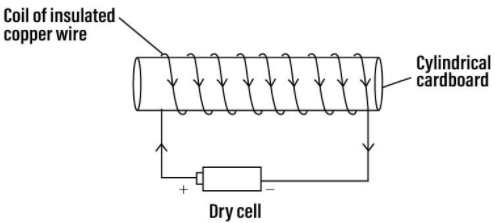When current is flowing through the solenoid, the magnetic field line pattern resemble exactly with those of a bar magnet with the fixed polarity North and South pole at its ends and it acquires the directive and attractive properties similar to bar magnet. Hence the current carrying solenoid behaves a bar magnet.
Use of current carrying solenoid: It is used to form a temporary magnet called electromagnet as well as permanent magnet.

Q.24. What are magnetic field lines? Justify the following statements
(a) Two magnetic field lines never intersect each other.     [CBSE 2014]
(b) Magnetic field lines are closed curves.     [CBSE 2015] [3 marks]
Ans. Magnetic field lines: It is defined as the path along which the unit North pole (imaginary) tends to move in a magnetic field if free to do so.
(a) The magnetic lines of force do not intersect (or cross) one another. If they do so then at the point of intersection, two drawn tangents at that point indicate that there will be two different directions of the same magnetic field, i.e. the compass needle points in two different directions which is not possible.
(b) Magnetic field lines are closed continuous curves. They emerge out from the north pole of a bar magnet and go into its south pole. Inside the magnet, they move, from south pole to north pole.

Q.1. Draw magnetic field lines produced around a current carrying straight conductor passing through a cardboard. Name, state and apply the rule to mark the direction of these field lines. How will the strength of the magnetic field change when the point where magnetic field to be determined is moved away from the straight conductor? Give reason to justify your answer.      [Allahabad 2019] [5 marks]
Ans.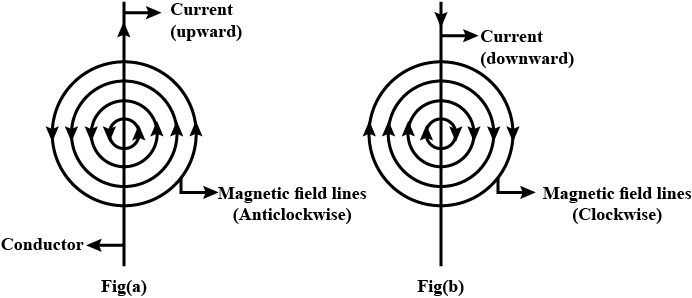Right-Hand Thumb Rule. This rule is used to find the direction of the magnetic field due to a straight current-carrying wire.
It states that if we hold the current-carrying conductor in the right hand in such a way that the thumb is stretched along the direction of the current, then the curly finger around the conductor represents the direction of the magnetic field produced by it.
Using a compass needles, we can determine the strength of the magnetic field at various points. If the needle is moved away from the straight wire carrying constant current, the deflection in the needle decreases. It implies that magnetic field strength decreases as the distance increases.

Q.2. (a) What are magnetic field lines? How is the direction of magnetic field at a point in a magnetic field determined using field lines?
(b) Two circular coils 'X' and 'Y' are placed close to each other. If the current in the coil 'X' is changed, will some current be induced in the coil 'Y'? Give reason.
(c) State 'Fleming's right hand rule.    [CBSE 2018C] [5 marks]
Ans. (a) It is defined as the path along which the hypothetical unit N-pole tends to move in a magnetic field, if free to do so.
By drawing a tangent at that point on the magnetic field lines one can find the direction of magnetic field at that point.
(b) Yes, of the current in coil X is changed, the magnetic field associated with it also changes around the coil 'Y' placed close to 'X'. This change in magnetic field lines linked with 'Y', according to Faraday law of electric magnetic induction, induces a current in the coil Y.
(c) Right-Hand Thumb Rule. This rule is used to find the direction of magnetic field due to a straight current carrying wire.
It states that if we hold the current carrying conductor in the right hand in such a way that the thumb is stretched along the direction of current, then the curly finger around the conductor represent the direction of magnetic field produced by it. This is known as right-hand thumb rule.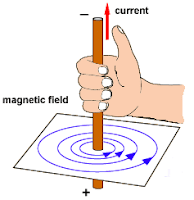Direction of Field Lines due to current carrying straight conductor as shown in figure.Q.3. (a) State Fleming’s left hand rule.
(b) Write the principle of working of an electric motor.
(c) Explain the function of the following parts of an electric motor.
(i) Armature
(ii) Brushes
(iii) Split ring    [CBSE 2018] [5 marks]
Ans.
(a) Fleming’s Left-Hand Rule: Stretch the thumb, forefinger and middle finger of the left hand such that they are mutually perpendicular to each other. If the forefinger pointed towards the direction of magnetic field and middle finger in the direction of current, then the thumb will indicate the direction of motion or force experienced by the conductor. It is to be applied only when the current and magnetic field, both are perpendicular to each other.
(b) Principle of an electric motor: It works on the principle of magnetic effect of current. When a current carrying conductor is placed perpendicular to the magnetic field, it experiences a force. The direction of this force is given by Fleming’s left hand rule.
(c) (i) Armature: It consists of a large number of turns of insulated current-carrying copper wires wound over a soft iron core and rotated about an axis perpendicular to a uniform magnetic field supplied by the two poles of permanent magnet.
(ii) Brushes: Two conducting stationary carbon or flexible metallic brushes constantly touches the revolving split rings or commutator. These brushes act as a contact between commutator and terminal battery.
(iii) Split ring: The split ring acts as a commutator in an electric motor. With the help of split ring, the direction of current through the coil is reversed after every half of its rotation and make the direction of currents in both the arms of rotating coil remains same. Therefore, the coil continues to rotate in the same direction.

Q.4. A current-carrying conductor is placed in a magnetic field. Now answer the following :
(i) List the factors on which the magnitude of force experienced by conductor depends.
(ii) When is the magnitude of this force maximum?
(iii) If initially this force was acting from right to left, how will the direction of force change, if :
(a) direction of magnetic field is reversed?
(b) direction of current is reversed?    [CBSE 2016] [5 marks]
Ans. (i) The magnitude of force experienced by the current-carrying conductor when placed in a magnetic field depends on current flowing, length of the conductor, the strength of magnetic field, orientation of conductor in the magnetic field.
(ii) Magnitude of force is maximum when current-carrying conductor is placed at right angles to the direction of magnetic field.
(iii) (a) Direction of force is reversed that is now the force acts from left to right.
(b) Direction of force is reversed that is now the force acts from left to right.

Q.5. In our daily life, we use two types of electric current whose current-time graphs are given in Fig. 13.29.
(i) Name the type of current in two cases.
(ii) Identify any one source for each type of current.
(iii) What is the frequency of current in case
(iv) in our country?
(v) Out of the two which one is used in transmitting electric power over long distances and why?    [CBSE 2011, 2014, 2015]. [5 marks]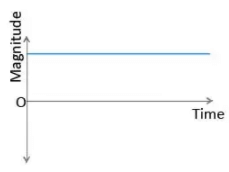Fig (a)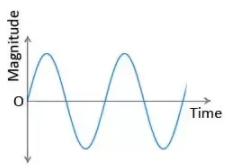Fig (b)
Ans.  (i) Current shown in Fig. (a) is direct current (D.C.) but current shown in Fig. (b) is an alternating current (A.C.).
(ii) A cell/battery produces D.C. but an A.C. generator produces A.C.
(iii) In India frequency of A.C. is 50 Hz.
(iv) A.C. is used in transmitting electric power over long distances. It is so because transmission loss of electric power can be minimised for A.C. by employing suitable transformers at generating stations and consuming centres.

Q.6. A student fixes a sheet of white paper on a drawing board. He places a bar magnet in the centre of it. He sprinkles some iron filings uniformly around the bar magnet. Then he taps the board gently.
Now answer the following questions :
(i) What does the student observe? Draw a diagram to illustrate your answer.
(ii) Why do the iron filings arrange in such a pattern?
(iii) What does the crowding of the iron filings at the ends of the magnet indicate?    [CBSE 2015]  [5 marks]

Ans.
(i) The student observes the magnetic field due to the given bar magnet. The pattern of the magnetic field is shown here.
(ii) There is a magnetic field around the given bar magnet. The iron filings experience a force due to the magnetic field and thus align themselves along the magnetic field lines.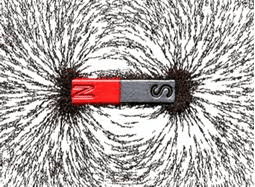(iii) The crowding of the iron filings at the ends of the magnet indicates the position of two magnetic poles N and S of a given bar magnet.

The document Previous Year Questions: Magnetic Effects of Current Notes | Study Science Class 10 - Class 10 is a part of the Class 10 Course Science Class 10.
All you need of Class 10 at this link: Class 10Use Code STAYHOME200 and get INR 200 additional OFF

## Science Class 10

107 videos|325 docs|162 tests

Track your progress, build streaks, highlight & save important lessons and more!

,

,

,

,

,

,

,

,

,

,

,

,

,

,

,

,

,

,

,

,

,

;